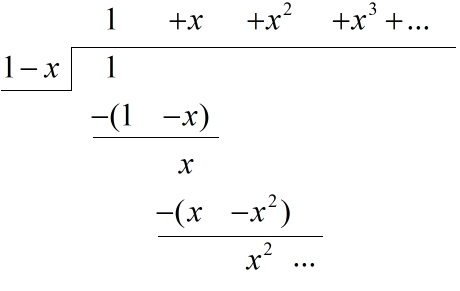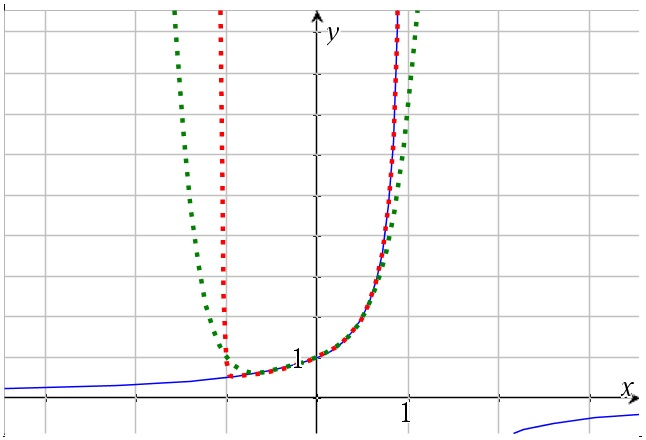# Power Series – an introduction

Up a level : Power Series
Next page : Mclaurin series - a first takeA power series is defined as$f(x) = {a_0} + {a_1}x + {a_2}{x^2} + {a_3}{x^3} + ... = \sum\limits_{k = 0}^\infty {{a_k}{x^k}}$

where the coefficients, ak, are constants. If all these constants above some numbers of terms are 0 then we have a finite power series, else we have an infinite power series. Finite power series are polynomials .

We define$\sum\limits_{k = 0}^\infty {{a_k}{x^k}} = \mathop {\lim }\limits_{n \to \infty} \sum\limits_{k = 0}^n {{a_k}{x^k}}$

If this limit exists then we say that the series converges to that limit, else we say that it diverges.

An infinite geometric series can be seen as a power series. If we have$f(x) = a + abx + a{b^2}{x^2} + a{b^3}{x^3} + ... = \sum\limits_{k = 0}^\infty {a{b^k}{x^k}}$

then the first term  u1 = a and the common ratio is r=bx. The sum of an infinite geometric series is${S_\infty } = \frac{{{u_1}}}{{1 - r}},\quad - 1 < r < 1$

I.e. it converges when r is between  –1 and 1. So for our series we get${S_\infty } = \frac{a}{{1 - bx}},\quad - 1 < bx < 1$

If we have a=1 and b=1 we get$\frac{1}{{1 - x}} = 1 + x + {x^2} + {x^3} + ... = \sum\limits_{k = 0}^\infty {{x^k}} ,\quad - 1 < x < 1$

This we could prove by  by the standard proof for the sum of a geometric series. We could also have found that by doing a polynomial division.  We have thatIf we assume we already have the answer we could simply multiply trough by 1-x. This gives us$\begin{gathered} (1 - x)(1 + x + {x^2} + {x^3} + ...) \hfill \\ \;{\kern 1pt} {\kern 1pt} = 1 + x + {x^2} + {x^3} + ... - x - {x^2} - {x^3} - ... \hfill \\ \;{\kern 1pt} {\kern 1pt} = 1,\quad - 1 < x < 1 \hfill \\ \end{gathered}$

The above is not a rigours proof, but it at least gives us a hint of that it is correct.

In the figure below the function and the series for six and 50 terms are plotted. We can clearly see that the series coverages to the function as values of x somewhat between –1 and 1.Up a level : Power Series
Next page : Mclaurin series - a first takeLast modified: Mar 3, 2019 @ 09:19